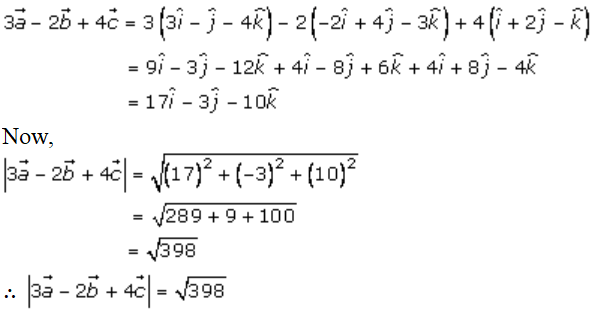# RD Sharma Solutions Class 12 Algebra Of Vectors Exercise 23.6

RD Sharma Solutions for Class 12 Maths Chapter 23 Algebra of Vectors Exercise 23.6 are provided here. Students who wish to develop a strong grip over the key concepts and methods of solving can refer to RD Sharma Solutions. These solutions are designed by subject experts in an easy-to-understand language, following a step-by-step procedure. Further, the solutions to this exercise can be accessed in PDF format, which can be downloaded from the link provided below.

## Download PDF of Rd Sharma Solution for Class 12 Maths Chapter 23 Exercise 6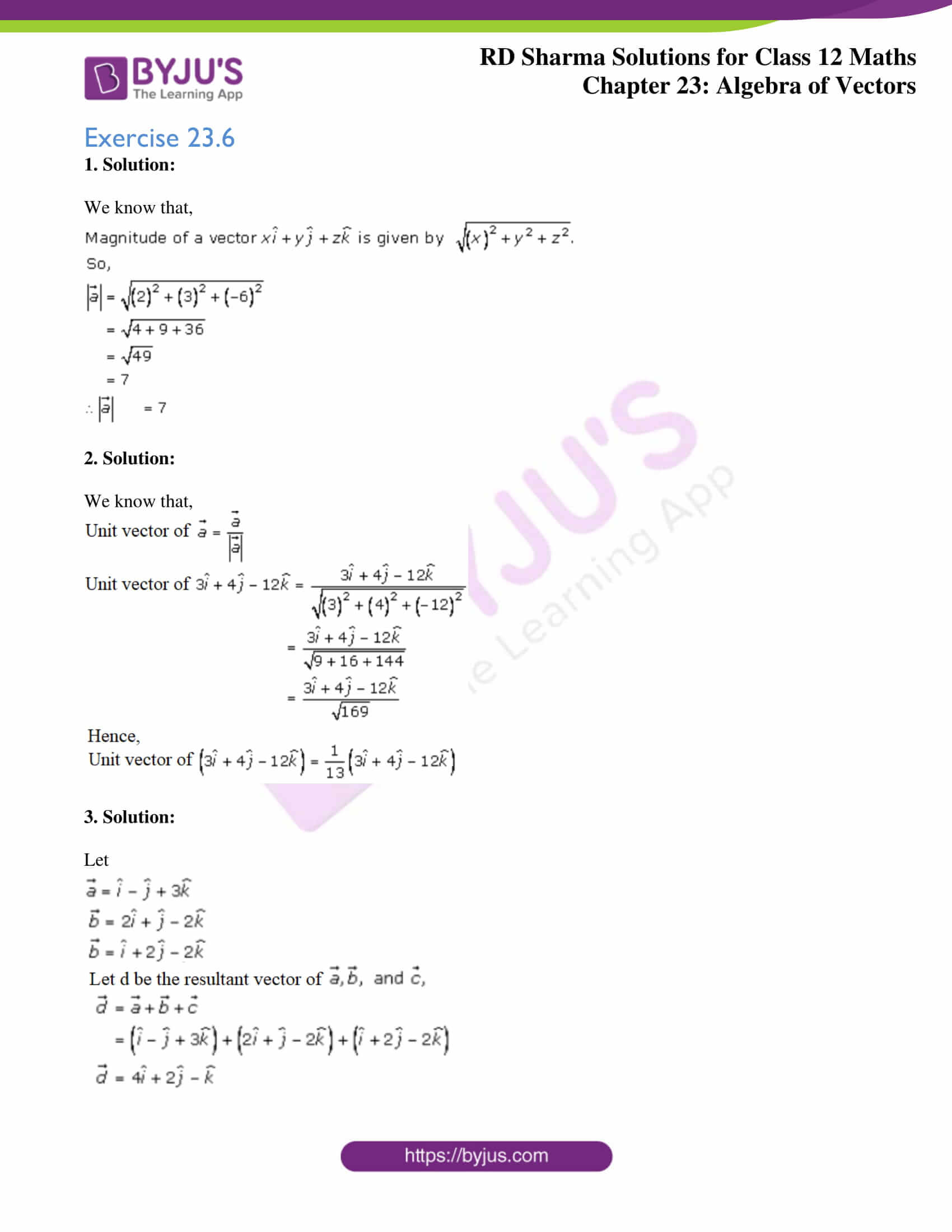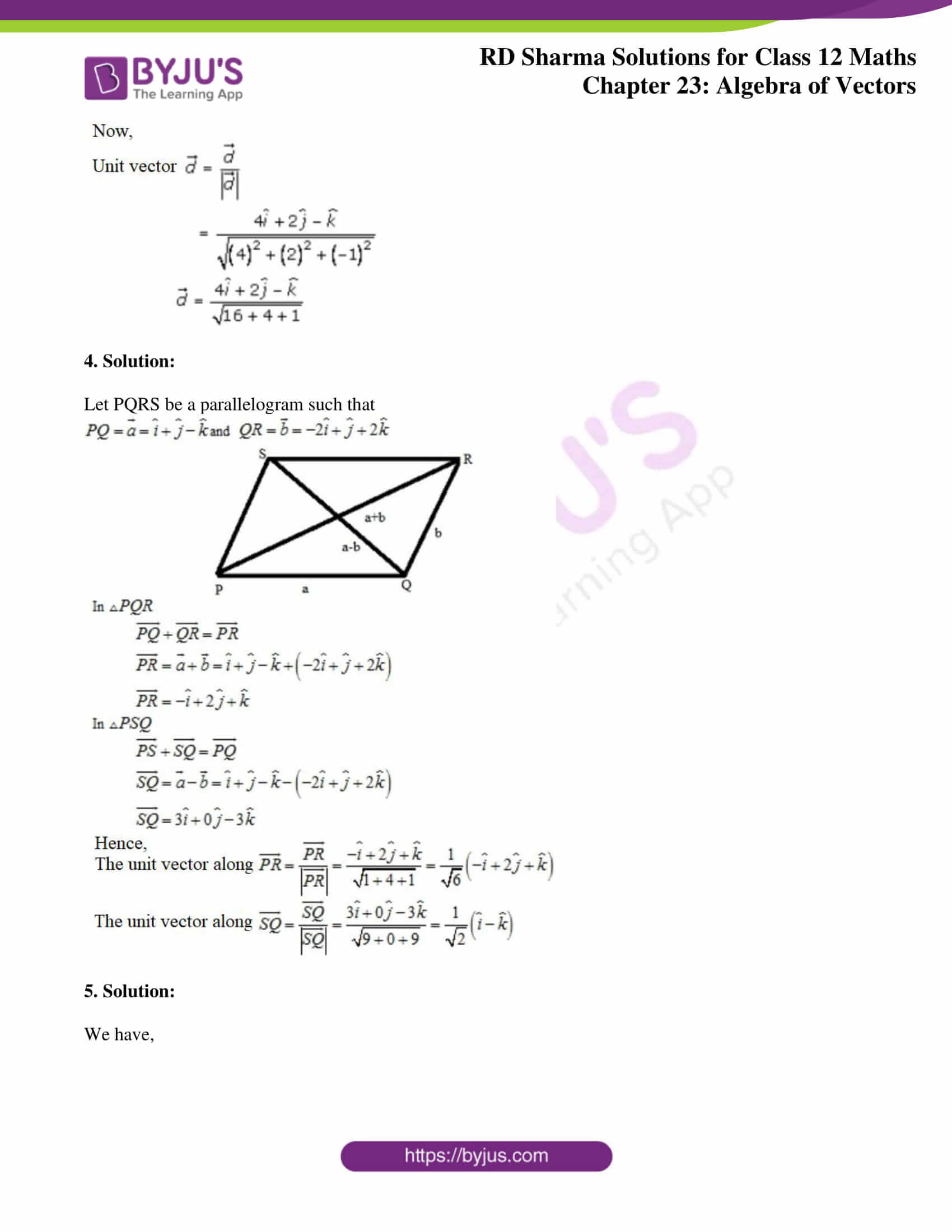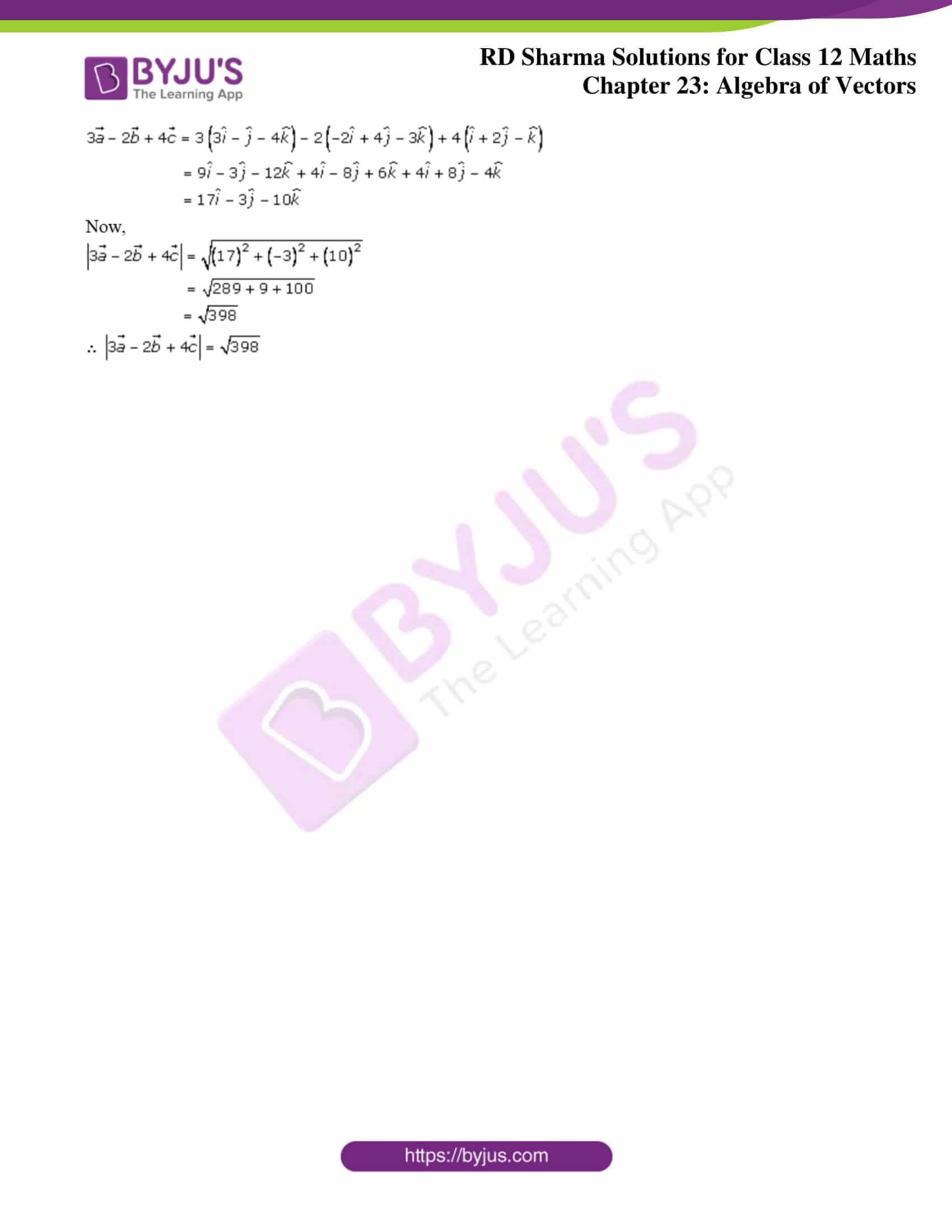### Access Answers for Rd Sharma Solution Class 12 Maths Chapter 23 Exercise 6

Exercise 23.6

1. Solution:

We know that,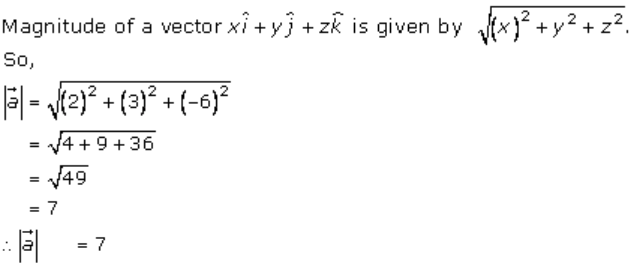2. Solution:

We know that,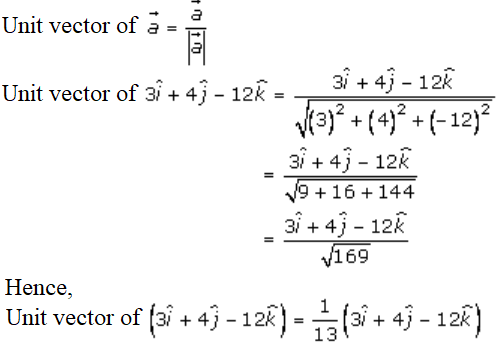3. Solution:

Let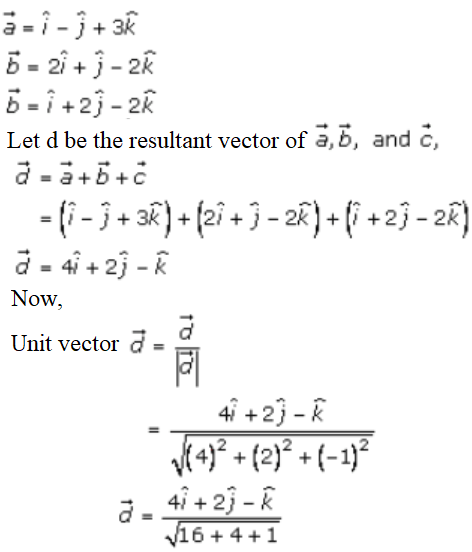4. Solution:

Let PQRS be a parallelogram such that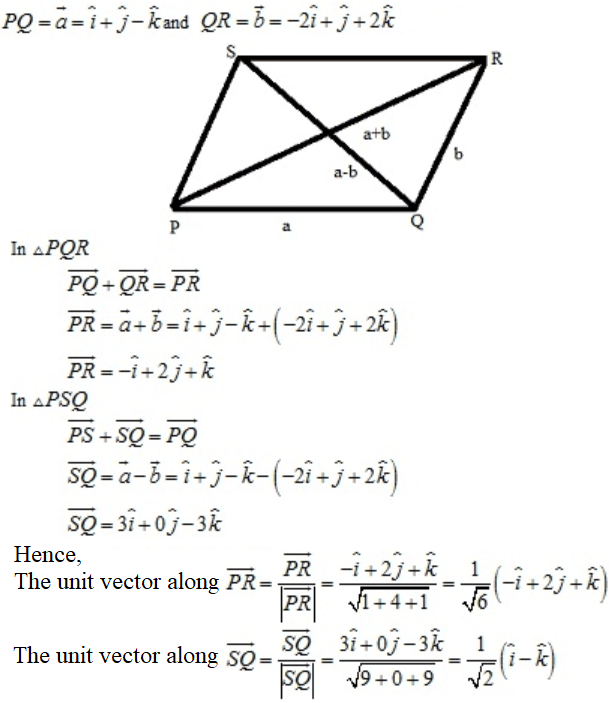5. Solution:

We have,